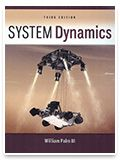Teach with MATLAB and Simulink

# "System Dynamics with Simscape" Curriculum Materials## Lecture Slides, MATLAB Code, and Models Using Simulink and Simscape

This downloadable package includes five sets of lecture slides introducing and applying Simscape to system dynamics problems in electromechanical, hydraulic, and thermal systems based on examples from Palm’s textbook. Each set of lecture slides is accompanied by a physical model developed with Simscape and a step-by-step guide on how to create it. More than 40 MATLAB and Simulink examples are also included. All materials were developed by Palm or under his supervision, based on his own course materials.### System Dynamics, 3rd Edition

By William J. Palm, III

Written for engineering students, this leading text introduces system dynamics and fully integrates MATLAB and Simulink examples throughout. The book includes discussion of the root locus and frequency response plots, among other methods for assessing system behavior in the time and frequency domains, as well as topics such as function discovery, parameter estimation, system identification techniques, motor performance evaluation, and system dynamics in everyday life.

• Chapter 1: Introduction
• Chapter 2: Dynamic Response and the Laplace Transform Method
• Chapter 3: Modeling of Rigid-Body Mechanical Systems
• Chapter 4: Spring and Damper Elements in Mechanical Systems
• Chapter 5: Block Diagrams, State-Variable Models and Simulation Methods
• Chapter 6: Electrical and Electromechanical Systems
• Chapter 7: Fluid and Thermal Systems
• Chapter 8: System Analysis in the Time Domain
• Chapter 9: System Analysis in the Frequency Domain
• Chapter 10: Introduction to Feedback Control Systems
• Chapter 11: Control System Design and the Root Locus Plot
• Chapter 12: Compensator Design and the Bode Plot
• Chapter 13: Vibration Applications
• Appendices
• Guide to Selected MATLAB Commands and Functions
• Fourier Series
• Developing Models from Data
• Introduction to MATLAB
• Numerical Methods

MathWorks and McGraw-Hill have developed a set of system dynamics models and lecture slides introducing Simscape to accompany William Palm’s System Dynamics text.